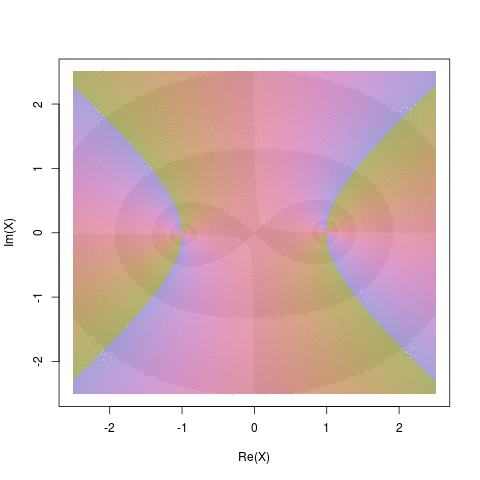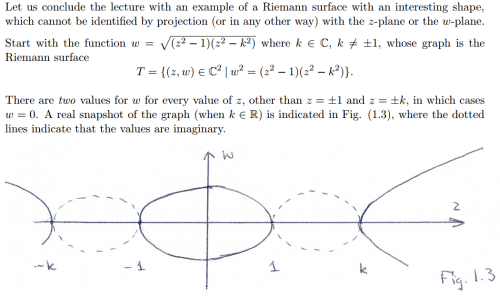Want to share your content on R-bloggers? click here if you have a blog, or here if you don't.√(x²−1)(x²−k²), k∈ℂ√(x²−1)(x²−k²).      x,k∈ℂ

(actually just going over the unit circle, not all of ℂ)

edit: hey, are these showing up as moving gif’s for you?

code:

```   require(animation)
source(wegert.R)    #where I define "plat" and "Z", standard for all Wegert plots
i = complex(imaginary=1)
hyperbola <- function(x,k=i) sqrt( (x^2-1) * (x^2-k^2) )
saveGIF({
for (param in 1:100/30) {
plat(Z, function(x) hyperbola(x,k=complex(argument=param)) )
}
}, movie.name="hyperbola", nmax=100)
```

more gifs: http://ge.tt/2Lh48dw1